# Topic 2 Multiple Choice Questions

All the following questions are from previous exams for Economics 103. They are duplicates of the questions found in the Topic sub-sections.

### Exercises 2.2

1. Consider the PPF diagram below.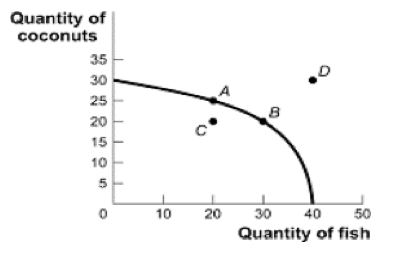Given the PPF illustrated, what is the opportunity cost of moving from B to A?

a) 5 coconuts.
b) 10 fish.
c) 5/10 fish
d) 10/5 coconuts.

The following TWO questions refer the diagram below, which illustrates the PPF for a producer of two goods, x and y.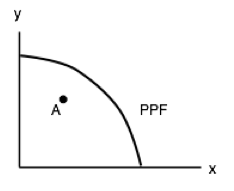2. Which of the following statements is TRUE?

I.    The marginal cost of producing x is higher at high levels of x than it is at low levels of x.
II.   The marginal cost of producing y is higher at high levels of y than it is at low levels of y.
III.  The marginal cost of producing both x and y is constant in the level of production.

a) I only.
b) II only.
c) III only.
d) I and II only.

3. If this economy is operating at point A, which of the following statements is TRUE?

I.   The opportunity cost of producing more x is zero.
II.  The opportunity cost of producing more y is zero.
III. Point A is inefficient.

a) III only.
b) I and II only.
c) I and III only.
d) I, II, and III.

The following TWO questions refer to the PPF diagram below.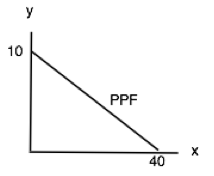4. What is the MARGINAL cost of producing good y?

a) 1/4 of a unit of x.
b) 1/4 of a unit of y.
c) 4 units of x.
d) 4 units of y.

5. What is the cost of producing FOUR units of good y?

a) 16 units of x.
b) 4 units of x.
c) 1/4 of a unit of x.
d) 40 units of x.

6. Consider a PPF drawn with x on the horizontal axis and y on the vertical axis. Which of the following concepts can be used to explain why this production possibility frontier could be flat at relatively lows levels of x and steep at relatively high levels of x?

a) Increasing marginal costs.
b) Scarcity
c) Sunk costs.

7. Which of the following concepts can be used to explain why production possibility frontiers slope downwards.

a) Scarcity
b) Sunk costs.
d) Increasing marginal costs.

### Exercises 2.3

1. The following question refers to the table below, which shows the maximum number of goods X and Y that producers A and B can produce in one day.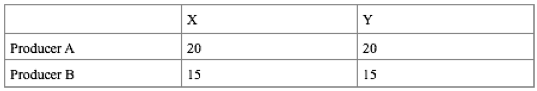Which of the following statements in TRUE?

a) Producer A has the comparative advantage in producing X.
b) Producer A has the comparative advantage in producing Y.
c) Producer B has the absolute advantage in producing X and Y.
d) No producer has the comparative advantage in producing either X or Y.

2. Consider the PPF diagram drawn below, for two countries that are free to trade with one another.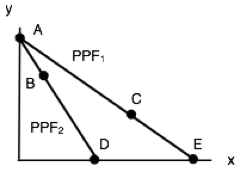Which of the following production combinations is/are INEFFICIENT?

I.   Country 1 produces at point C and country 2 produces at point D.
II.  Country 1 produces at point E and country 2 produces point at B.
III. Country 1 produces at point E and country 2 produces at point A.

a) II only.
b) I only.
c) I and II only.
d) I, II and III.

3. The diagram below illustrates the PPFs for two countries that produce wine and cheese. With no trade, country 1 produces at point A on its PPF and country 2 produces at point B.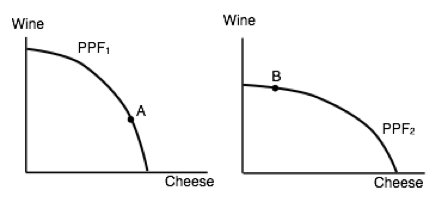Assume that the two countries now begin to trade with one another. Which of the following will NOT occur (relative to the case with no trade).

a) Country 1 will produce less cheese.
b) Country 2 will export wine.
c) Country 1 will import cheese.
d) Country 2 will produce more cheese.

4. Which of the following statements about production and trade is FALSE?

I.  If a country has an absolute advantage in producing a good, then it also has the comparative advantage in the production of that good.
II. Rich countries will generally have the comparative advantage in the production of all goods.
III. If a country has the absolute advantage in the production of a good, then this country will be made better off by specializing in the production of that good.a) I only.
b) I and II only.
c) I, II and III.
d) III only.

The following THREE questions refer to the diagram below, which illustrates the PPFs for two countries who are free to trade.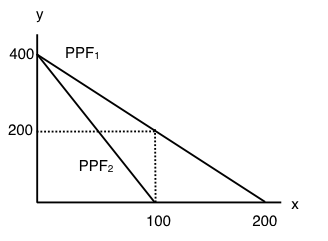5. What is the marginal opportunity cost (MC) of producing good x in each country?

a) 2 units of good y in country 1 and 4 units of good y in country 2.
b) 1/2 a unit of good y in country 1 and 1/4 of a unit of good y in country 2.
c) 2 units of good y in country 1 and 1/4 of a unit of good y in country 2.
d) 1/2 a unit of good y in country 1 and 4 units of good y in country 2.

6. What is the marginal opportunity cost (MC) of producing good y in each country?

a) 2 units of good x in country 1 and 4 units of good x in country 2.
b) 1/2 a unit of good x in country 1 and 1/4 of a unit of good x in country 2.
c) 2 units of good x in country 1 and 1/4 of a unit of good x in country 2.
d) 1/2 a unit of good x in country 1 and 4 units of good x in country 2.

7. Suppose that aggregate production of x across the two countries is equal to 100 (that is, country one’s production of x plus country two’s production of x equals 100 units). If these 100 units of x are being produced efficiently, then aggregate production of y will equal:

a) 200 units of y.
b) 400 units of y.
c) 600 units of y.
d) 800 units of y.

8. The diagram below illustrates the identical PPFs of two countries.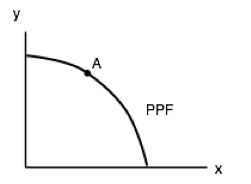Initially, there is no trade allowed between the two countries, and each country produces at point A. If trade is opened up, which of the following will occur?

I.   Country 1 will export coal to country 2.
II.  Country 2 will produce more clothing.
III. Country 1 will produce less coal.

a) I and II only.
b) III only.
c) II and III only.
d) None of the above.

9. The table below shows the maximum amounts of coffee and salmon that Brazil and British Colombia can produce if they just produce one good.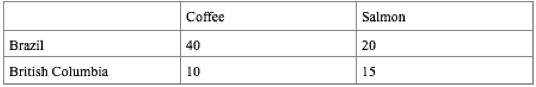Assuming constant marginal costs:

a) Brazil has a comparative advantage in coffee production
b) In Brazil, the marginal cost of salmon production is 2 units of coffee.
c) In BC, the marginal cost of coffee production is 1½ units of salmon.
d) All of the above are correct.

10. The diagram below illustrates the PPFs for two countries that produce two goods. The two countries are free to trade with one another.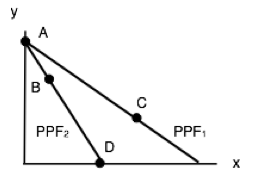Which of the following production combinations are efficient?

a) Country 1 is at point C; country 2 is at point D.
b) Country 1 is at point A; country 2 is at point B.
c) Country 1 is at point C; country 2 is at point A.
d) All of the above are efficient.